## Programming Challenges

### PC2 & PC3

If you downloaded the file, you’ll need to point R’s load() command at the correct file location on your machine. You can also use url() within load() to point at a web address. Here I’ve chosen to demonstrate with group 8’s data.

load(url("https://communitydata.cc/~ads/teaching/2019/stats/data/week_02/group_02.RData"))

ls() # shows me what's available
##  "d"

A clarifying point that came up in class: if you’re writing your own RMarkdown script, you will need to load the dataset explicitly (not just open the file with RStudio). When RMarkdown tries to “knit” the .Rmd file into HTML or whatever, it is as if you are running the entire contents of the .Rmd in an entirely new RStudio environment. This means if you don’t load something explicitly RStudio will not know it’s there!

### PC4

min(d)
##  -5.550215
max(d)
##  15415.83
mean(d)
##  738.7643
median(d)
##  7.278341
var(d)
##  6380870
sd(d)
##  2526.038
IQR(d)
##  87.33717

### PC5

Graphing using R’s built-in functions:

hist(d)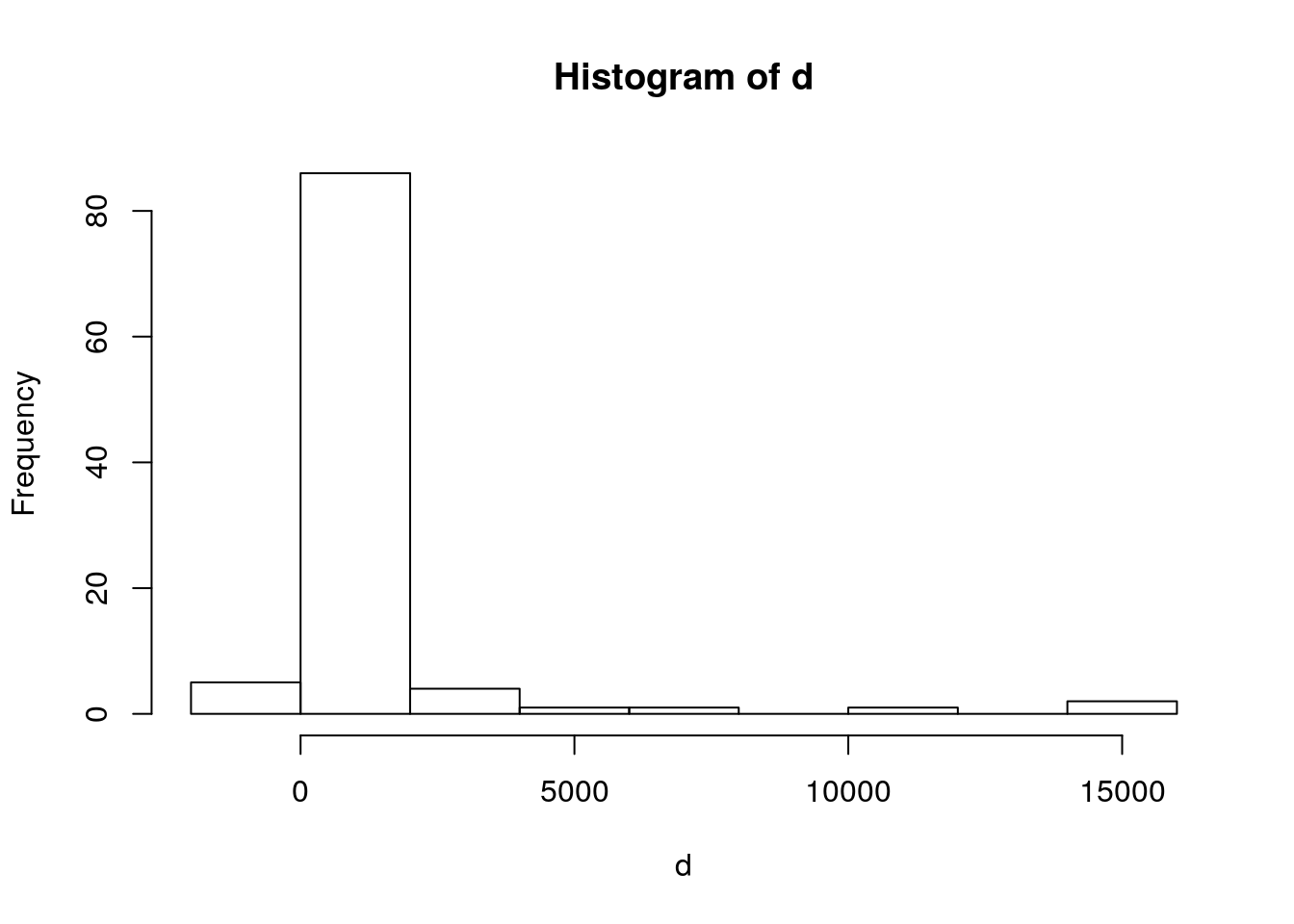boxplot(d)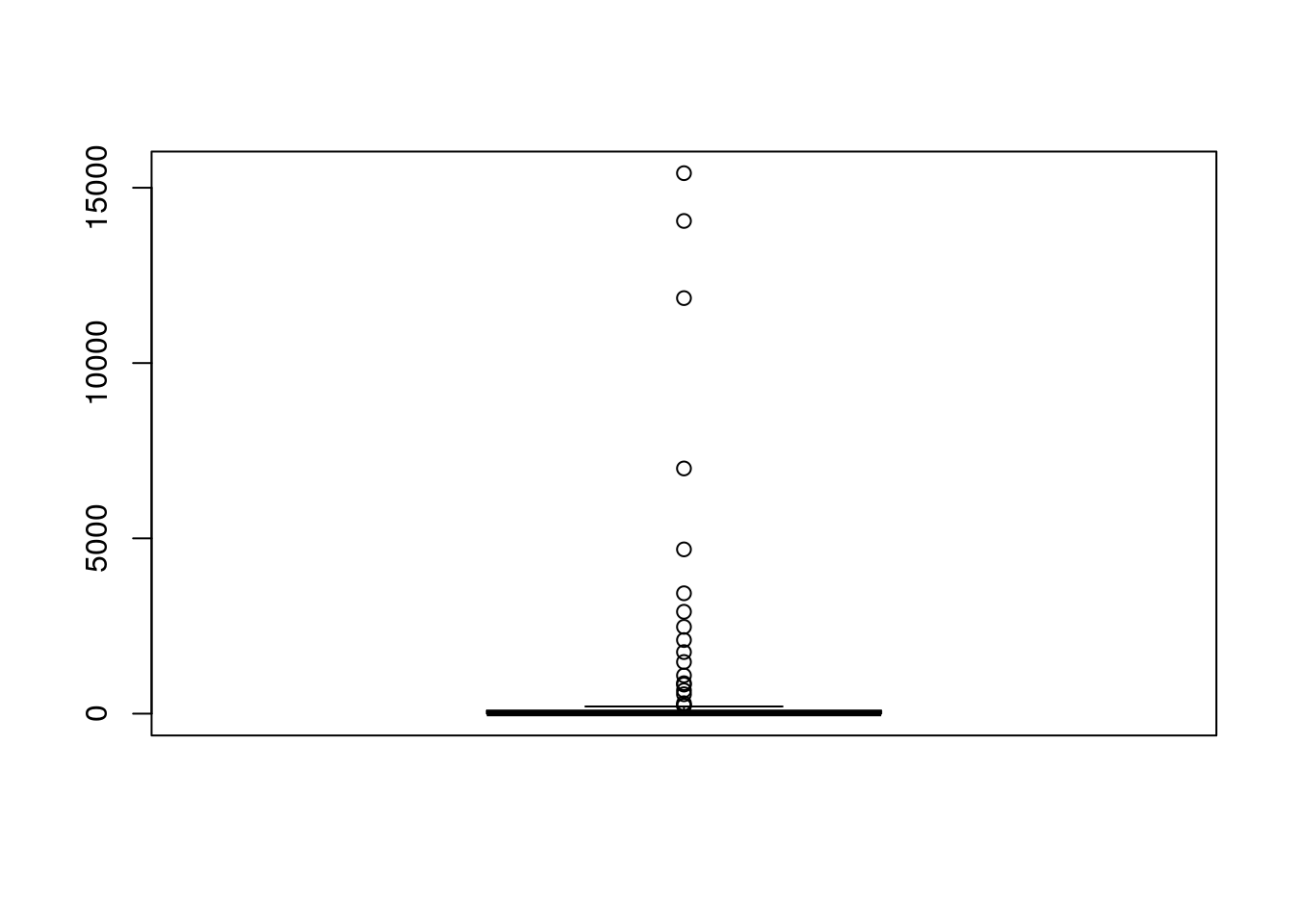Next week, we will start learning to use more of the ggplot2 package (make sure it’s installed first). I’ll reproduce the historgram and boxplot in ggplot2 and leave these examples here for future reference. Graphing a single vector in ggplot2 is a bit weird, so this looks a little different than the examples from the R lecture:

library(ggplot2)
p <- ggplot() + aes(d)
p + geom_histogram()
## stat_bin() using bins = 30. Pick better value with binwidth.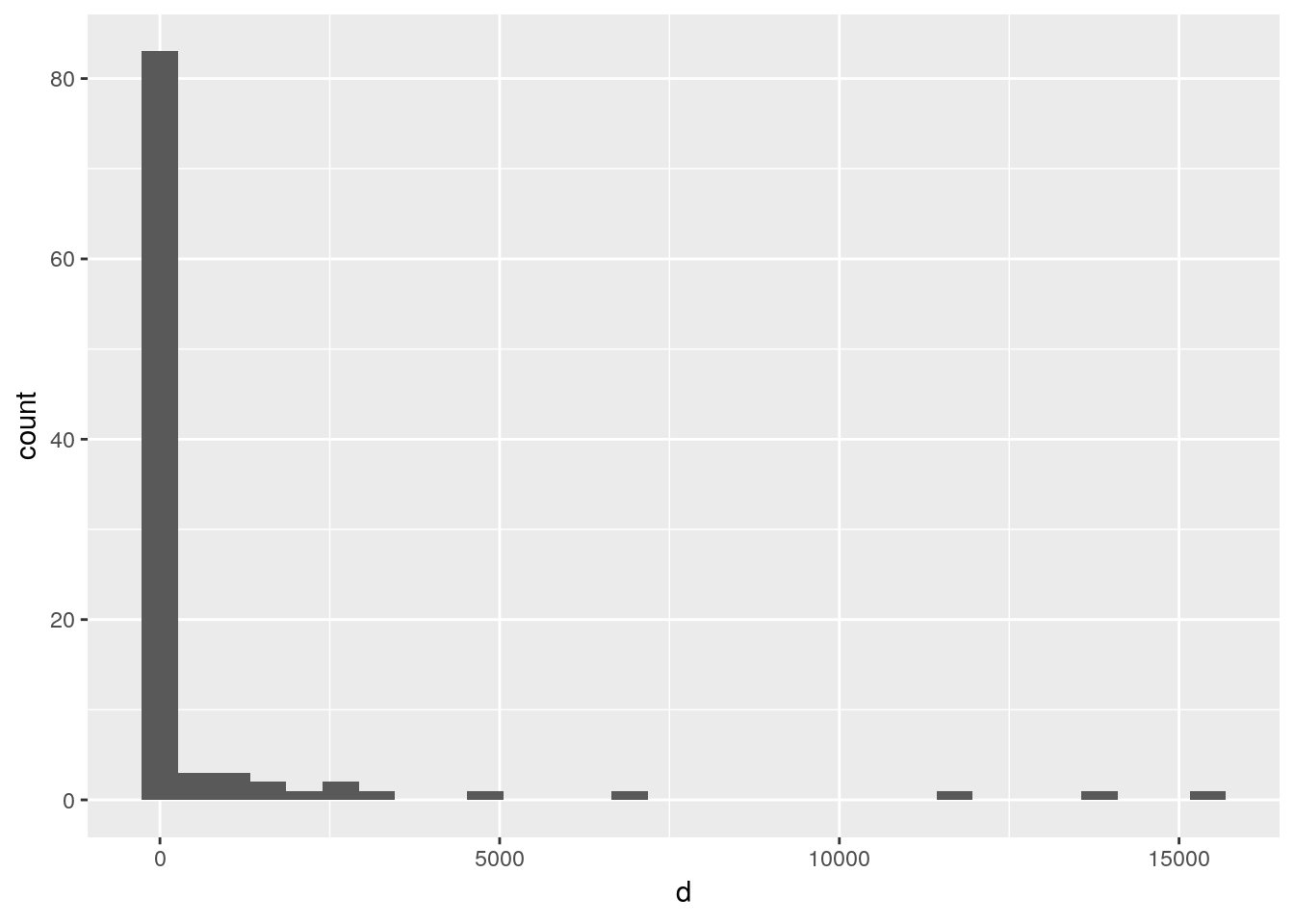p + geom_boxplot(aes(x="", y=d))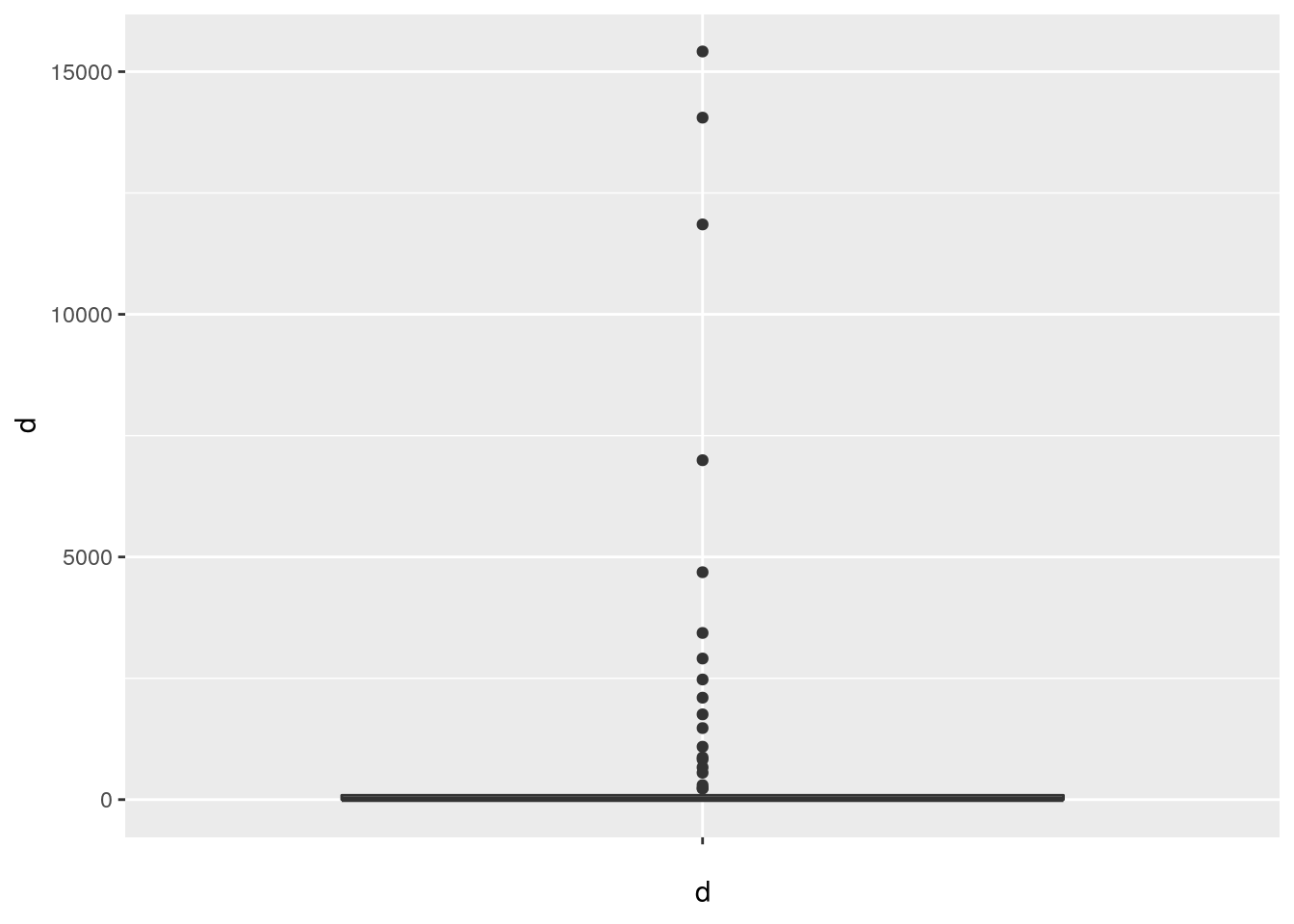During today’s class a question came up about changing the axis limits, so here is an examples of how that would look for the histogram in ggplot2. Note that the coord_cartesian layer only adjusts the “zoom” of the image on the axes. The axes themselves are generated in the call to geom_histogram which looks at the range of the underlying data and (by default) distributes that into 30 “bins.” See the ggplot2 documentation for to learn how to adjust the number of bins directly. Also, feel free to fiddle around with the values passed to the xlim and ylim arguments below to see what happens:

p + geom_histogram() + coord_cartesian(xlim=c(-50,17000), ylim=c(0,100))
## stat_bin() using bins = 30. Pick better value with binwidth.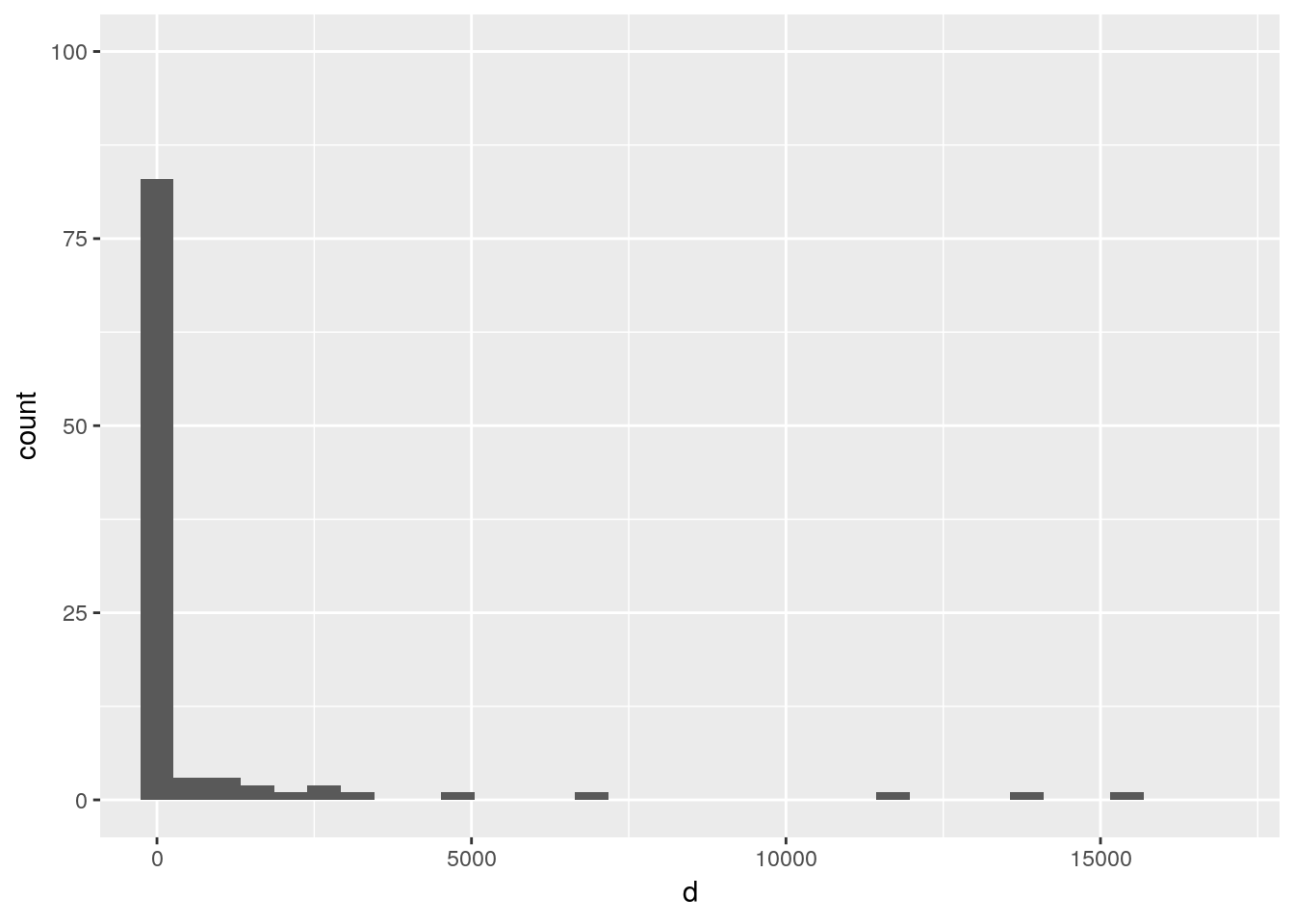### PC6

dd <- d # You might create a new object to work with just for clarity.
dd[d < 0] <- NA

mean(dd, na.rm=T) # R can understand "T" in place of TRUE
##  777.7835
sd(dd, na.rm=T)
##  2586.407

### PC7

d.log <- log1p(dd) # Note: I use log1p() because some values are very close to zero

mean(d.log, na.rm=T)
##  3.163984
median(d.log, na.rm=T)
##  2.258885
sd(d.log, na.rm=T)
##  2.655523
hist(d.log)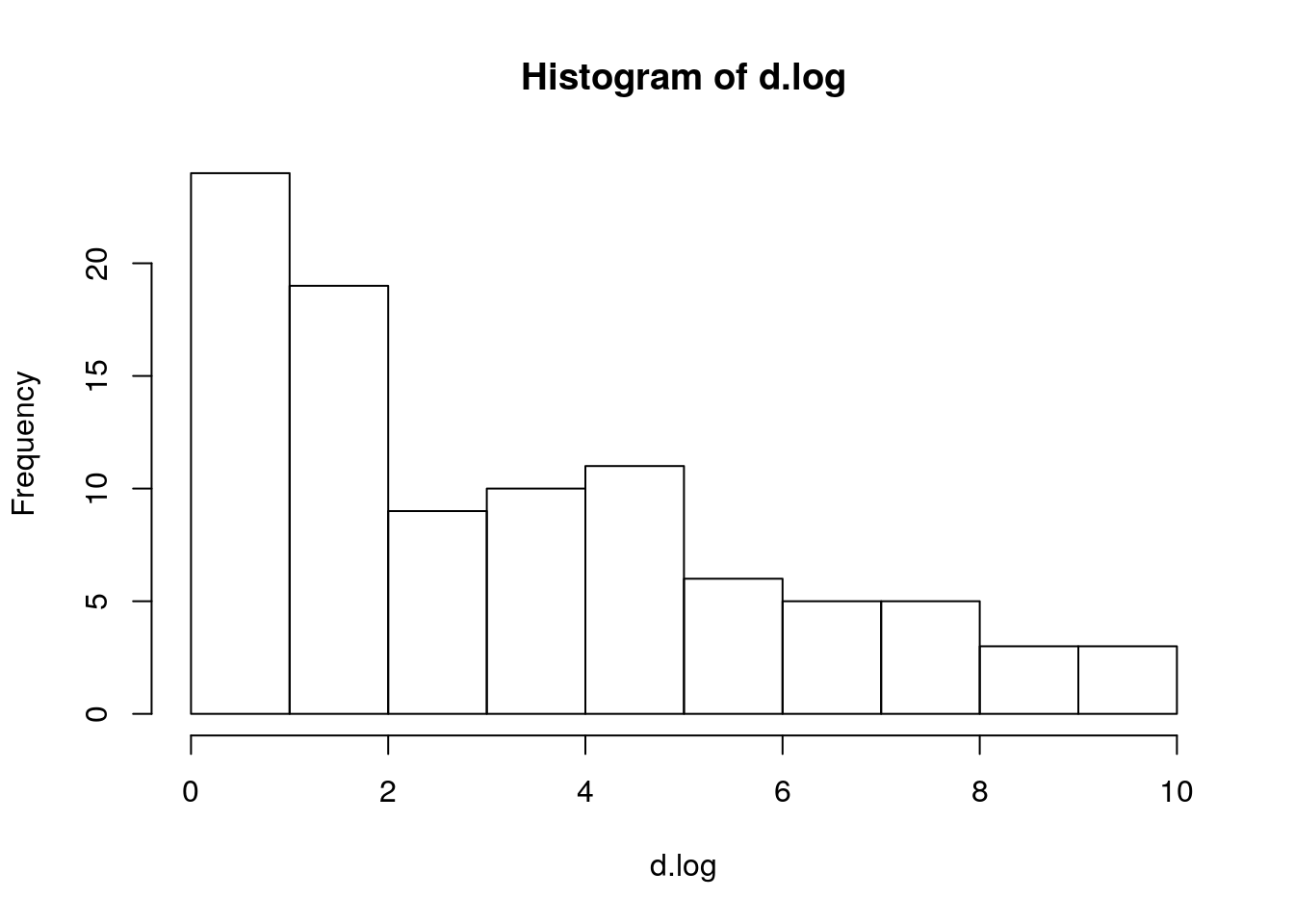boxplot(d.log)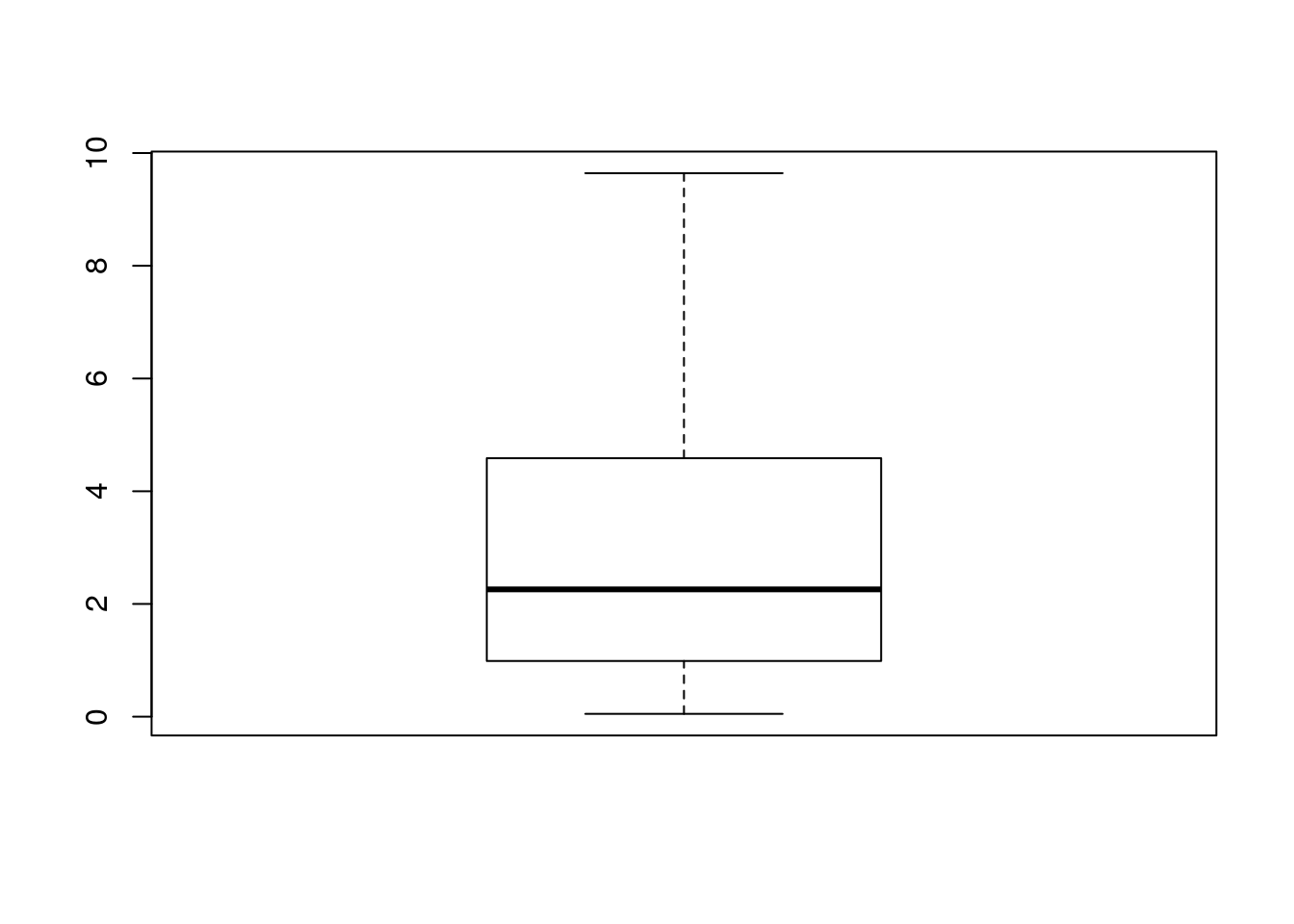## Statistical Questions

Please get in touch with any questions or clarifications. I realize these solutions are a bit concise. I’my happy to clarify the underlying logic.

### SQ 1 (2.12)

1. By the addition rule: $$P(no~missed~days) = 1 - (0.25 + 0.15 + 0.28) = 0.32$$
2. $$P(1~miss~or~less) = P(no~misses) + P(1~miss)$$ $$= 0.32 + 0.25 = 0.57$$
3. $$P(at~least~1~miss) = P(1~miss) + P(2~misses) + P(\geq 3~misses)$$ $$= 1 - P(no~misses) = 1 - 0.32 = 0.68$$
4. Assume (foolishly!) that the absences are independent. This allows us to use the multiplication rule:
$$P(neither~miss~any) = P(no~misses) × P(no~misses) = 0.32*2 = 0.1024$$
5. Again, assume that the absences are independent and use the multiplication rule:
$$P(both~miss~some) = P(at~least~1~miss) × P(at~least~1 miss) = 0.68*2 = 0.4624$$
6. Siblings are likely to get each other sick, so the independence assumption is not sound.

### SQ 2 (2.20)

This one is all about calculating the conditional probabilities from a contingency table:

1. $$P(man~or~partner~has~blue~eyes) = (108 + 114 - 78) / 204 = 0.7059$$
2. $$P(partner~with~blue~eyes | man~with~blue~eyes) = 78 / 114 = 0.6842$$
3. $$P(partner~with~blue~eyes | man~with~brown~eyes) = 19 / 54 = 0.3519$$ $$P(partner~with~blue~eyes | man~with~green~eyes) = 11 / 36 = 0.3056$$
4. Partner eye color does not appear independent within this sample. Blue-eyed partners are more common for blue-eyed individuals than other eye colors.

### SQ 3 (2.26)

More conditional probabilities, this time calculating a compound probability:

$$P(identical|females) = \frac{P(identical~and~females)}{P(females)}$$ $$= \frac{0.15}{0.15 + 0.175}$$ $$= 0.46$$

(A decision tree may also useful here)

### SQ 4 (2.32)

1. Once you have one person’s birthday, the odds of the second person have the same birthday are: $$P(first~two~share~birthday) = \frac{1}{365} = 0.0027$$

2. This one is more challenging! I find it easier to think about by focusing on the probability that the first two don’t share a birthday, followed by the probability that the next person doesn’t share a birthday either:

$$P(at~least~two~share~birthday) = 1-P(none~of~three~share~birthday)$$ $$=1-P(first~two~don't~share) \times P(third~doesn't~share~either)$$
$$=1-(\frac{364}{365}) \times (\frac{363}{365}) =0.0082$$

#### Bonus pop-quiz question from class:

If I offered you a choice between a bet on the flip of a fair coin or whether any two people in our (25 person) class shares a birthday, what should you choose?

I like the following approach to this question:

Consider that 25 people can be combined into pairs $${25 \choose 2}$$ ways, which is equal to $$\frac{25 \times 24}{2} = 300$$ (see the Wikipedia article on binomial coefficients for more on that).

Generalizing the logic from part b of the previous problem, I assume that each of these possible pairings are independent and thus each one has a probability $$P = (\frac{364}{365})$$ of producing a non-matched set of birthdays.

Put everything together, employ the multiplication rule and you get the following: $P(any~match) = 1 - P(no~matches)$
$P(no~matches) = (\frac{364}{365})^{300}$
Let’s let R take it from here:

1-((364/365)^300) 
##  0.5609078

I’d take the coin flip if I were you!

### SQ 5 (2.38)

1. First, the average fee ($$F$$) per passenger is the expected value of the fees per passenger:

$$E(Fee~per~passenger) = \0(0.54) + \25(0.34) + \60(0.12)$$ $$= \0 + \8.5 + \7.2 = \15.70$$

To calculate the standard deviation, we need to calculate the square root of the variance. To find the variance, we take the deviance at each fee level, multiply that by the probability (frequency) of the fee level, and calculate the sum:

Deviance: $$(F - E(F))^2$$
No baggage: $$(0-15.70)^2 = 246.49$$
1 bag: $$(25-15.70)^2 = 86.49$$
2 bags: $$(60-15.70)^2 = 1962.49$$

Deviance multiplied by probability: $$(F - E(F))^2 \times P(F)$$
No baggage: $$246.49 \times 0.54 = 133.10$$
1 bag: $$86.49 \times 0.34 = 29.41$$
2 bags: $$1962.49 \times 0.12 = 235.50$$

Variance ($$V$$): $$\398.01$$
Standard deviation ($$SD$$): $$19.95$$

1. Assume independence of individual passengers (probably wrong, but maybe not catastrophic?):

For 120 passengers:
$$E(Revenue) = 120 \times 15.70 = \1,884$$
$$V = 120 \times 398.01 = \47,761.20$$
$$SD = \sqrt{47761.20} = \218.54$$

### SQ 6 (2.44)

1. Right skewed, with a median somewhere around $35-$50,000. There’s a long tail out to the right.
2. Addition rule: $$P(Income \lt \50k) = 2.2 + 4.7 + 15.5 + 18.3 + 21.2 = 62.2\%$$
3. Assume that income and gender are independent and the general multiplication rule holds:
$$P(Income \lt \50k~and~female) = P(Income \lt \50k) \times P(female) = 0.622 \times 0.41 = 0.255$$
4. It seems that the assumption was not so great since the actual proportion of women with incomes less than $$\50k$$ was a lot higher than we would expect based on the multiplied conditional probabilities in part c.

## Empirical Paper Questions

### EQ 1

1. “Top” U.S. political blogs.
2. 155 blogs selected for the study in summer 2008.

### EQ 2

$$P(sole~authored | left~perspective) = \frac{17}{17+37+10} = 0.266$$

### EQ 3

$$P(right~perspective|large~scale~collab.) = \frac{4}{10+4} = 0.286$$

### EQ 4

The conditional probabilites provide some evidence that left wing blogs were more collaborative than right wing blogs. That said, a substantial proportion of left blogs are sole-authored, so it’s not clear (to me at least) that left blogs are all that likely to be collaborative in their own right. In addition, the number of large scale collaborative blogs overall is not very big (consider Jeremy’s point that if you had 4 heads out of 14 coin flips you might not be that surprised!). Nevertheless, the conditional probabilities do suggest that right blogs are highly unlikely to be large collaborations. A formal hypothesis test such as the $$\chi^2$$ test in the paper is arguably more convincing, but the assumptions underlying the null hypothesis may be a little funny (we can discuss this more in a few weeks).

### EQ 5

I’ll be curious to hear what you think!